# 机器学习菜鸟的学习笔记（12）

• Python
• 机器学习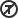原创

### 线性回归的实现

#### 文件读取

xProfit in \$10,000s, y代表了Population of City in 10,000s

 with open(filename, 'r') as f:


 plt.plot(data_x, data_y, 'rx', 10)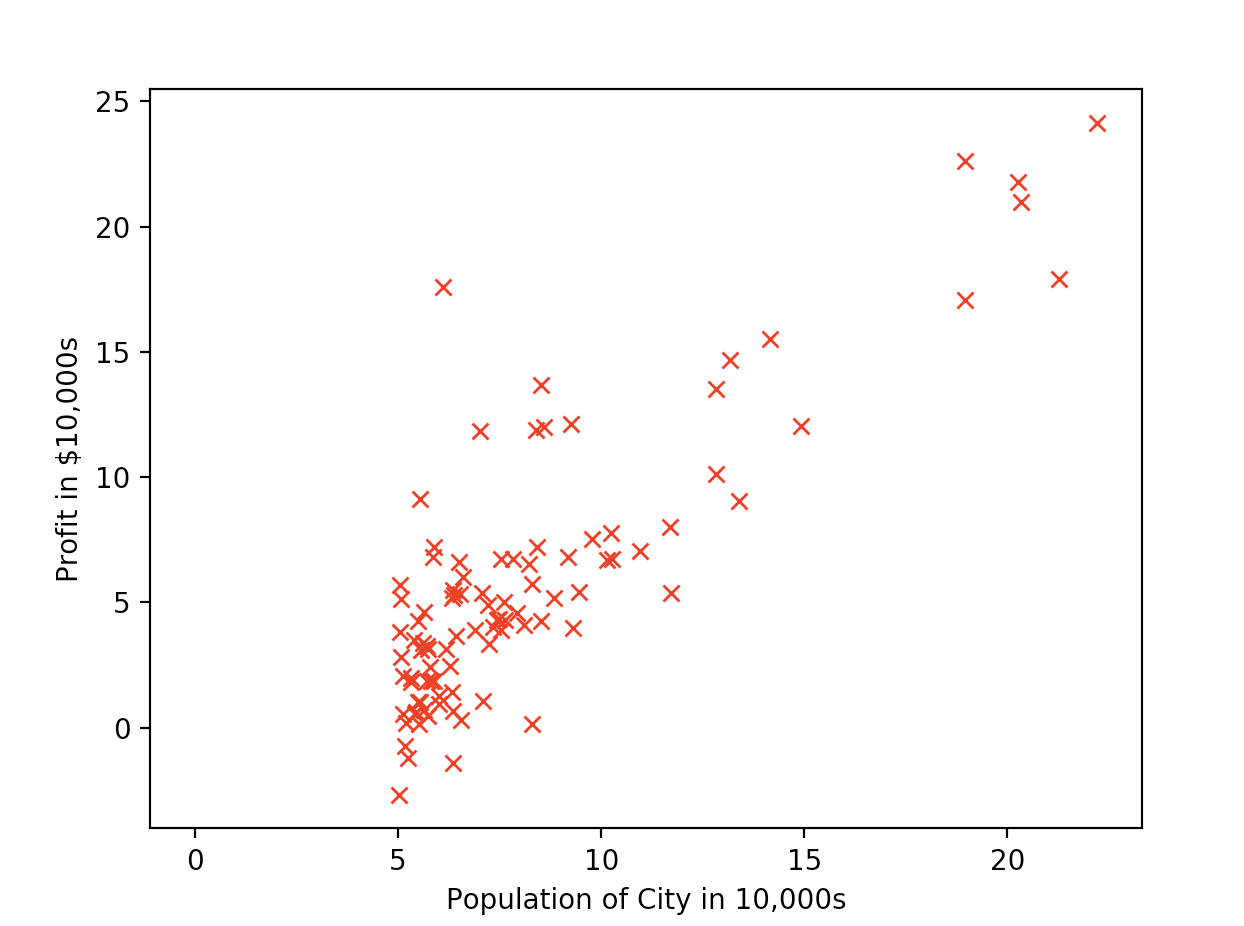#### 转成矩阵

data_x = [[1, row] for row in data_x]
matrix_x = np.mat(data_x)


#### 损失函数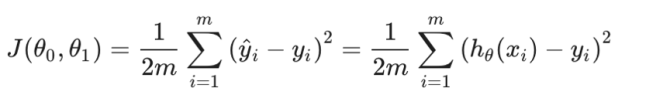# compute suqare errors
sqrErrors = np.power((data_x * theta_in - data_y), 2).sum()
# compute cost
J = sqrErrors / (2 * len(data_y))


#### 梯度下降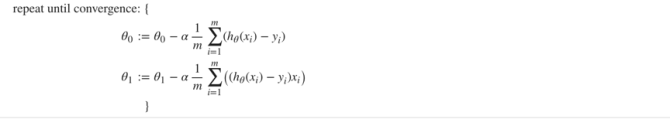J_history = np.zeros([nums_ite, 1])
for ite in range(1, nums_ite):
theta = theta - select_alpha / m * data_x.T * (data_x * theta - data_y)
J_history[ite] = compute_cost(data_x, data_y, theta)


#### 结果展示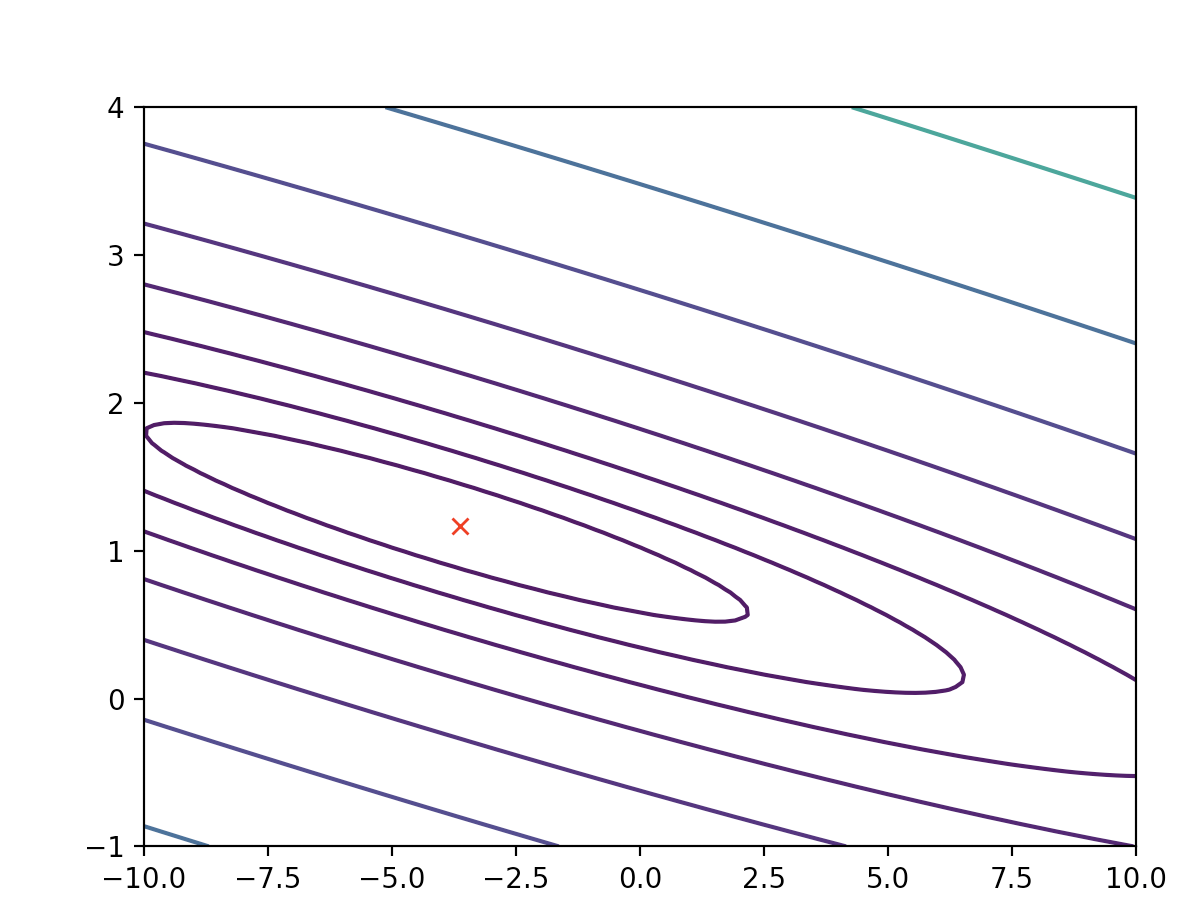1

0

3